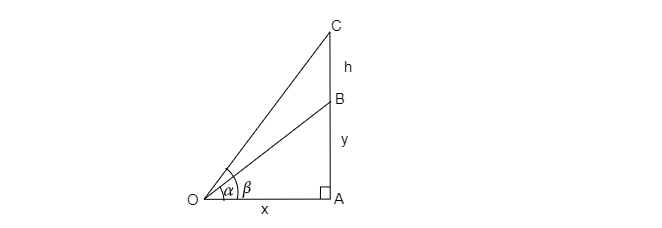# A vertical tower stands on a horizontal plane and is surmounted by a vertical flagstaff of height $h$. At a point on the plane, the angles of elevation of the bottom and the top of the flag staff are $\alpha$ and $\beta$, respectively. Then find the height of the tower.

Given: A vertical tower stands on a horizontal plane and is surmounted by a vertical flagstaff of height $h$. At a point on the plane, the angles of elevation of the bottom and the top of the flag staff are $\alpha$ and $\beta$, respectively.

To do: To find the height of the tower.

Solution:Let height be $y \vartriangle OAC$

$tan\beta =\frac{CA}{OA}$

$tan\beta =\frac{y+h}{x}$   $( y+h)=CA=AB+BC,\ Let OA=x$

$x=( \frac{y+h}{tan\beta})$

Consider $\vartriangle OAB$

$tan\alpha =\frac{y}{x}$

$x=\frac{y}{tan\alpha }$
$\frac{y}{tan\alpha }=\frac{y+h}{tan\beta}$

$y.tan\beta =y.tan\alpha +h.tan\alpha$

$y.tan\beta −y.tan\alpha=h.tan\alpha$

$y(tan\beta −tan\alpha )=h.tan\alpha$

$y=\frac{h.tan\alpha}{tan\beta −tan\alpha }$

Updated on: 10-Oct-2022

21 Views